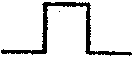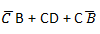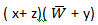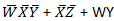# In the circuit shown, the switch S is open for a long time and is closed at t=0. The current i(t) for t ≥0+ is

1.   i(t)=0.5-0.125e-1000tA

2.   i(t)=1.5-0.125e-1000tA

3.   i(t)=0.5-0.5e-1000tA

4.  i(t)=0.375e-1000tA

4

i(t)=0.5-0.125e-1000tA

Explanation :
No Explanation available for this question

# In the circuit shown in figure, value of input p goes from 0→1 and that of goes from 1→0. Which output forms shown in figure represents the output under a static hazard conditions

1.

2.

3.

4.

4Explanation :
No Explanation available for this question

# In the circuit shown below, the initial charge on the capacitor is 2.5 mC, with the voltage polarity as indicated. The switch is closed at time t =0. The current i(t) at a time t after the switch is closed is

1.   i(t)=15 exp(-2 x 103 t)A

2.   i(t)=5 exp(-2 x 103 t)A

3.   i(t)=10 exp(-2 x 103 t)A

4.   i(t)=-5 exp(-2 x 103 t)A

4

i(t)=15 exp(-2 x 103 t)A

Explanation :
No Explanation available for this question

# The maximized expression for the given K-map is (X: don’t care)

1.

2.

3.

4.

4Explanation :
No Explanation available for this question

# In figures shown below x2 x1 x0 will be 1’s complement of A2 A1 A0 if

1.  Y = 0

2.  Y =1

3.  Y =  = , =

4.  Y = A0 = A1 = A2

4

Y =1

Explanation :
No Explanation available for this question

# For the parallel RLC circuit, which one of the following statements IS Not correct

1.  The bandwidth of the circuit decreases if R is increased

2.  The bandwidth of the circuit remains same if L is increased

3.  At resonance, input impedance is a real quantity

4.  At resonance, the magnitude of input impedance attains its minimum value

4

At resonance, the magnitude of input impedance attains its minimum value

Explanation :
No Explanation available for this question

# The circuit of a gate in the resistor transistor logic (RTL) family shown in the given figure is a/an

1.  AND gate

2.  OR gate

3.  NAND gate

4.  NOR gate

4

NAND gate

Explanation :
No Explanation available for this question

# K-map for a Boolean function is shown in the figure. The function would be

1.

2.

3.

3Explanation :
No Explanation available for this question

# The circuit below implements a filter between the input current i1 and the output voltage v0. Assume that the opamp is ideal. The filter implemented is a

1.   Low pass filter

2.   Band pass filter

3.  Band stop filter

4.   High pass filter

4

High pass filter

Explanation :
No Explanation available for this question

# For the K-map shown in figure, the minimized function in SOP form is:

1.

2.

3.

4.

4